U.S. Department of Transportation
1200 New Jersey Avenue, SE
Washington, DC 20590
202-366-4000

Federal Highway Administration Research and Technology
Coordinating, Developing, and Delivering Highway Transportation Innovations

 REPORT This report is an archived publication and may contain dated technical, contact, and link information
 Publication Number:  FHWA-HRT-12-030    Date:  August 2012
 Publication Number: FHWA-HRT-12-030 Date: August 2012

# Estimation of Key PCC, Base, Subbase, and Pavement Engineering Properties From Routine Tests and Physical Characteristics

PDF files can be viewed with the Acrobat® Reader®

# CHAPTER 5. MODEL DEVELOPMENT (7)

Figure 141 through figure 143 show the sensitivity of this model to CMC, w/c ratio, and age, respectively. These trends are all reasonable. Figure 141 and figure 142 show the change in compressive strength at two ages, 28 days and 1 year, which are almost at the lower and upper bounds of ages included in this model. The plot in figure 143 can be considered a strength gain curve for typical unit weight and w/c ratios used in mix designs.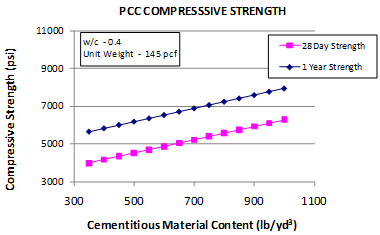#### Figure 141. Graph. Short-term cylinder compressive strength sensitivity to CMC.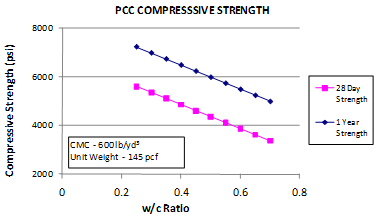#### Figure 142. Graph. Short-term cylinder compressive strength sensitivity to w/c ratio.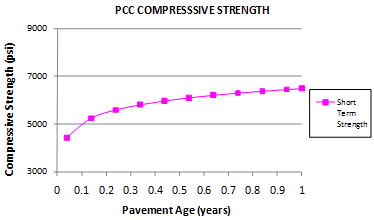### Compressive Strength Model 3: Short-Term Core Strength Model

The core strength data in the LTPP database were used for this model. While the materials and test ages are similar to the short-term cylinder strength model, the compressive strength of the cores is representative of the consolidation and quality of construction in the field. An initial comparison of core versus cylinder strengths was performed to determine if there was a significant difference in two strength values. Data were matched by section and age. Data were grouped in several age categories so that strength comparisons could be made at corresponding ages. Generally, each category up to 56 days was grouped for ages of ±3 days. For ages close to 6 months to 1 year, the results were grouped for ages ±30 days. The ages at which strength test results were common to both cores and cylinders were 14 days, 21 days, 28 days, 35 days, 41 days, and 1 year.

The comparison showed that there was no significant difference between strength values determined from core or cylinder tests. The paired t-test results shown in table 25 indicate that there is no significant difference between the two strengths (P < t-critical). Figure 144, which has a trend line forced to zero intercept, shows the same results. Note that a trend line with a non-zero intercept produces a higher R2 (0.67), which is consistent with the Pearson correlation value of 0.82 presented in table 25.

In the development of this model, parameters similar to the cylinder strength model were evaluated. In addition, the effect of curing was considered with greater attention. However, curing did not prove to be a significant variable. As this model attempts to predict the strength up to 1 year in age, the variable accounting for age was treated in a hierarchical fashion.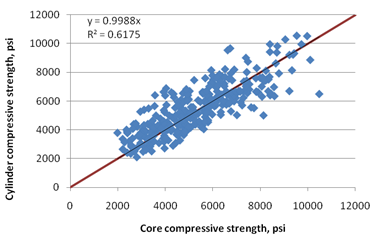#### Table 25. Paired t-test results for comparison of core and cylinder strengths in SPS data.

 Parameter Core Cylinder Mean 5345.3 5472.3 Variance 3,307,974.59 3,003,561.77 Observations 312 312 Pearson correlation 0.82 Hypothesized mean difference 0 DF 311 t-Stat -2.11 P(T ≤ t) one-tail 0.02 t-critical one-tail 1.65 P(T ≤ t) two-tail 0.04 t-critical two-tail 1.97

This model was established as shown in figure 145.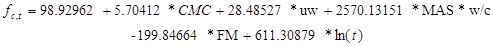#### Figure 145. Equation. Prediction model 3 for fc,t.

Where:

fc,t = Compressive strength at age t years, psi.

CMC = Cementitious materials content, lb/yd3.

uw = Unit weight, lb/ft3.

MAS = Maximum aggregate size, inch.

w/c = Water to cementitious materials ratio.

FM = Fineness modulus of fine aggregate.

t = Short-term age in years.

The regression statistics for this model are presented in table 26. The model was developed using 294 points, and the prediction has an R2 value of 67.6 percent and an RMSE value of 1,122 psi. Table 27 provides details of the range of data used to develop the model. Figure 146 and figure 147 show the predicted versus measured plot and the residual plot, respectively. Figure 148through figure 153 show the sensitivity of this model to CMC, unit weight, MAS, w/c ratio, FM, and age, respectively.

#### Table 26. Regression statistics for short-term core strength model.

 Variable DF Estimate Standard Error t-Value Pr > |t| VIF Intercept 1 98.92962 1,544.34064 0.06 0.949 0 Cementitious 1 5.70412 0.36589 15.59 < 0.0001 1.23548 Unit weight 1 28.48527 10.59672 2.69 0.0076 1.0182 (MAS) × (w/c ratio) 1 2,570.13151 538.267 -4.77 < 0.0001 1.2201 Fineness modulus (FM) 1 -199.84664 120.68288 -1.66 0.0988 1.01426 Ln(age) 1 611.30879 45.08962 13.56 < 0.0001 1.00026

The model statistics for table 26 are as follows:

• RMSE = 1,122 psi.
• R2 = 0.6761 percent.
• N = 294.

#### Table 27. Range of data used for short-term core strength model.

 Parameter Minimum Maximum Average w/c ratio 0.27 0.69 0.42 Cementitious content 376 999 670 Unit weight 120 163 144 MAS 0.375 1.000 0.683 FM 2.50 4.37 3.05 Pavement age 0.0380 2.2160 0.4230 Compressive strength 1,990 11,350 5,596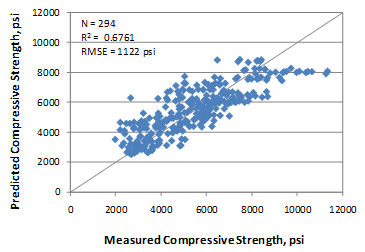#### Figure 146. Graph. Predicted versus measured for short-term core compressive strength model.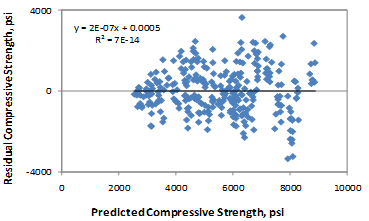#### Figure 147. Graph. Residual errors for short-term core compressive strength model.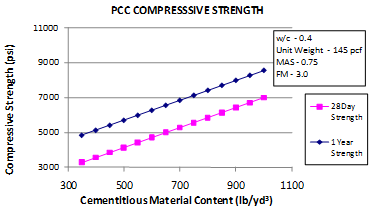#### Figure 148. Graph. Short-term core compressive strength sensitivity to CMC.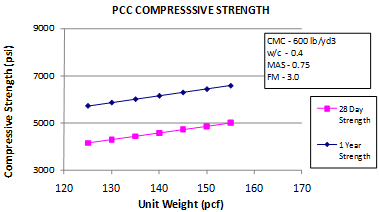#### Figure 149. Graph. Short-term core compressive strength sensitivity to unit weight.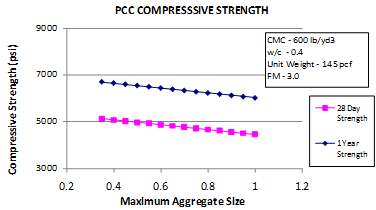#### Figure 150. Graph. Short-term core compressive strength sensitivity to MAS.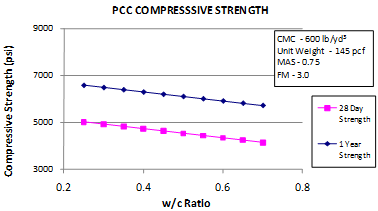#### Figure 151. Graph. Short-term core compressive strength sensitivity to w/c ratio.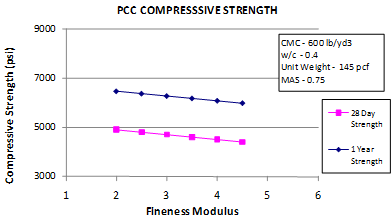#### Figure 152. Graph. Short-term core compressive strength sensitivity to fine aggregate FM.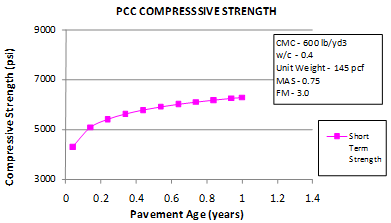#### Figure 153. Graph. Short-term core compressive strength sensitivity to age.

Federal Highway Administration | 1200 New Jersey Avenue, SE | Washington, DC 20590 | 202-366-4000
Turner-Fairbank Highway Research Center | 6300 Georgetown Pike | McLean, VA | 22101